# The UNIVARIATE Procedure

### Example 4.34 Comparing Weibull Quantile Plots

This example compares the use of three-parameter and two-parameter Weibull Q-Q plots for the failure times in months for 48 integrated circuits. The times are assumed to follow a Weibull distribution. The following statements save the failure times as the values of the variable Time in the data set Failures:

data Failures;
input Time @@;
label Time = 'Time in Months';
datalines;
29.42 32.14 30.58 27.50 26.08 29.06 25.10 31.34
29.14 33.96 30.64 27.32 29.86 26.28 29.68 33.76
29.32 30.82 27.26 27.92 30.92 24.64 32.90 35.46
30.28 28.36 25.86 31.36 25.26 36.32 28.58 28.88
26.72 27.42 29.02 27.54 31.60 33.46 26.78 27.82
29.18 27.94 27.66 26.42 31.00 26.64 31.44 32.52
;


If no assumption is made about the parameters of this distribution, you can use the WEIBULL option to request a three-parameter Weibull plot. As in the previous example, you can visually estimate the shape parameter c by requesting plots for different values of c and choosing the value of c that linearizes the point pattern. Alternatively, you can request a maximum likelihood estimate for c, as illustrated in the following statements:

title 'Three-Parameter Weibull Q-Q Plot for Failure Times';
ods graphics on;
proc univariate data=Failures noprint;
qqplot Time / weibull(c=est theta=est sigma=est)
square
href     = 0.5 1 1.5 2
vref     = 25 27.5 30 32.5 35
odstitle = title;
run;


Note: When using the WEIBULL option, you must either specify a list of values for the Weibull shape parameter c with the C= option or specify C=EST.

Output 4.34.1 displays the plot for the estimated value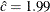. The reference line corresponds to the estimated values for the threshold and scale parameters of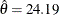and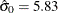, respectively.

Output 4.34.1: Three-Parameter Weibull Q-Q Plot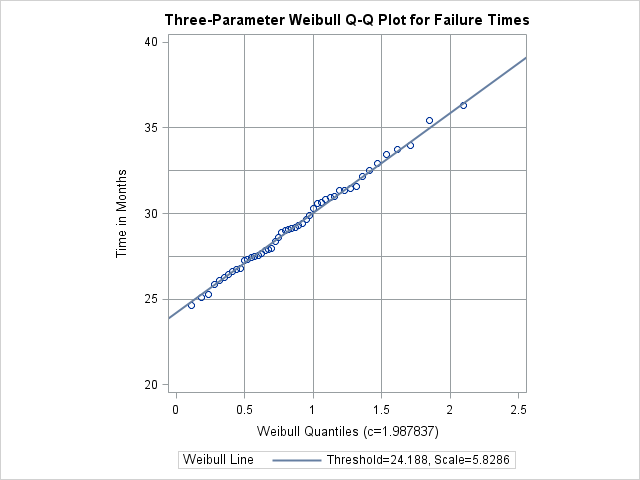Now, suppose it is known that the circuit lifetime is at least 24 months. The following statements use the known threshold value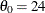to produce the two-parameter Weibull Q-Q plot shown in Output 4.31.4:

title 'Two-Parameter Weibull Q-Q Plot for Failure Times';
proc univariate data=Failures noprint;
qqplot Time / weibull(theta=24 c=est sigma=est)
square
vref     = 25 to 35 by 2.5
href     = 0.5 to 2.0 by 0.5
odstitle = title;
run;


The reference line is based on maximum likelihood estimates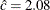and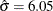.

Output 4.34.2: Two-Parameter Weibull Q-Q Plot for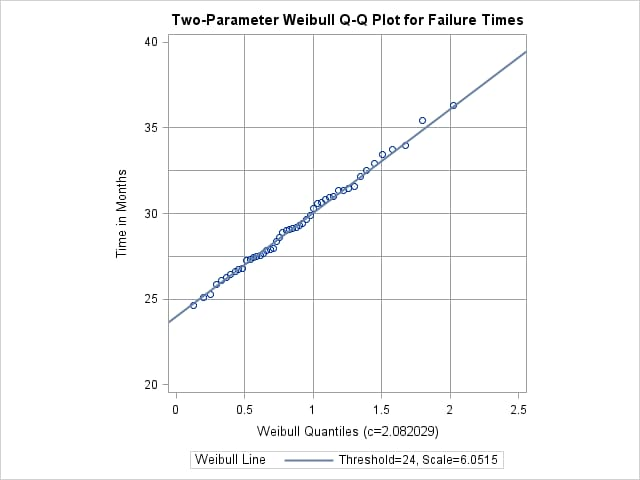A sample program for this example, uniex19.sas, is available in the SAS Sample Library for Base SAS software.